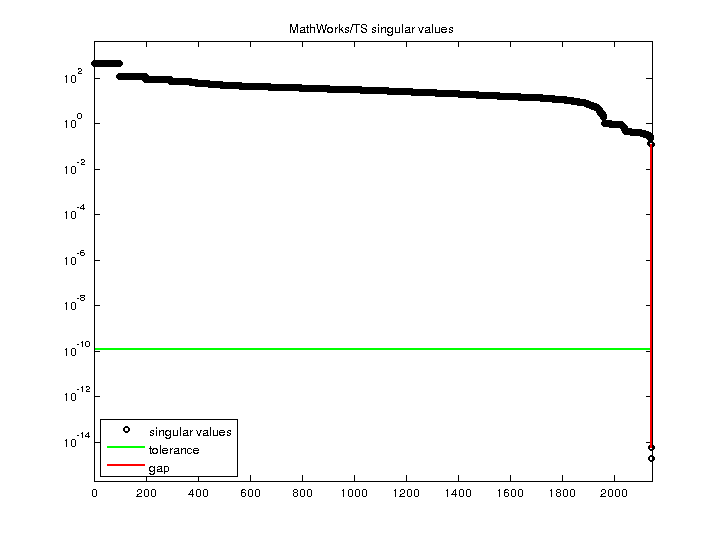Matrix: MathWorks/TS

Description: x=A\b triggers a bug in MATLAB R2009a and earlier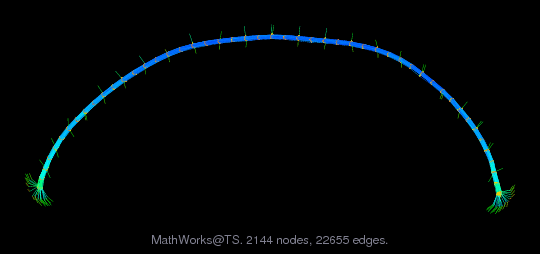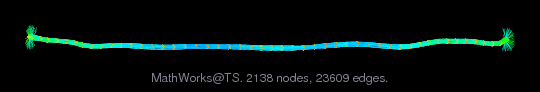(bipartite graph drawing) (graph drawing of A+A')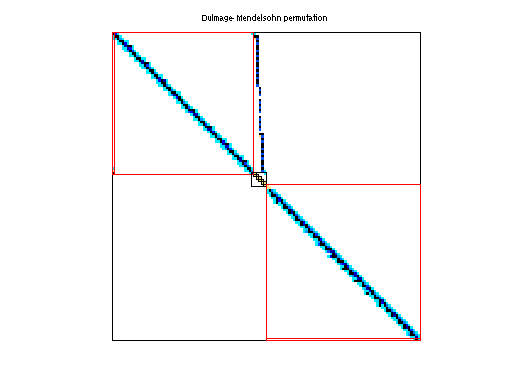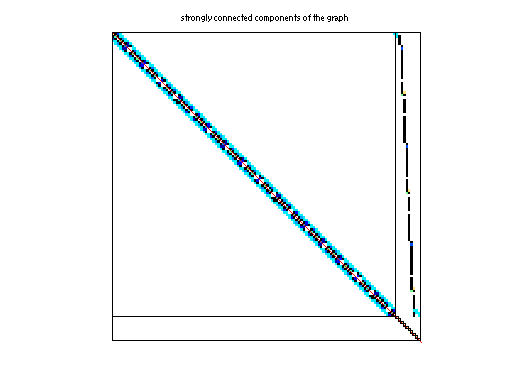• Matrix group: MathWorks
• download as a MATLAB mat-file, file size: 108 KB. Use UFget(2256) or UFget('MathWorks/TS') in MATLAB.

 Matrix properties number of rows 2,142 number of columns 2,142 nonzeros 45,262 structural full rank? no structural rank 2,140 # of blocks from dmperm 92 # strongly connected comp. 183 explicit zero entries 0 nonzero pattern symmetry 95% numeric value symmetry 4% type real structure unsymmetric Cholesky candidate? no positive definite? no

 author P. Quillen editor T. Davis date 2009 kind counter-example problem 2D/3D problem? no

 Additional fields size and type b sparse 2142-by-1

Notes:

```This is a structurally singular matrix that triggers a bug in the
2-by-2 pivoting strategy in UMFPACK versions 4.1 through 5.3.
The bug thus exists in MATLAB R2006b through R2009a.  It was
fixed in UMFPACK 5.4 and MATLAB R2009b.  Contributed by Pat Quillen,
The MathWorks, Inc.  The sprank(A) and rank(A) are 2140.
```

 Ordering statistics: result nnz(chol(P*(A+A'+s*I)*P')) with AMD 73,792 Cholesky flop count 2.8e+06 nnz(L+U), no partial pivoting, with AMD 145,442 nnz(V) for QR, upper bound nnz(L) for LU, with COLAMD 43,575 nnz(R) for QR, upper bound nnz(U) for LU, with COLAMD 86,695

 SVD-based statistics: norm(A) 443.42 min(svd(A)) 1.96114e-15 cond(A) 2.26103e+17 rank(A) 2,140 sprank(A)-rank(A) 0 null space dimension 2 full numerical rank? no singular value gap 2.05462e+13

 singular values (MAT file): click here SVD method used: s = svd (full (A)) ; status: ok